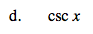### Home > CALC > Chapter Ch1 > Lesson 1.4.2 > Problem1-154

1-154.
1. If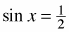and if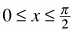, then without a calculator evaluate: Homework Help ✎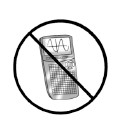1. cos x

2. tan x

3. sec x

4. csc x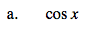$\text{If sin is }\frac{\text{opposite}}{\text{hypotenuse}}\text{ then you know that the hypotenuse is a multiple of 2 and the}$

opposite side is half that. What kind of special right triangle fulfills those conditions?$\frac{\sqrt{3}}{2}$

$\text{tan}(x)=\frac{\text{sin}(x)}{\text{cos}(x)}$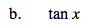$\text{sec}(x)=\frac{1}{\text{cos}(x)}$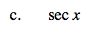$\text{csc}(x)=\frac{1}{\text{sin}(x)}$